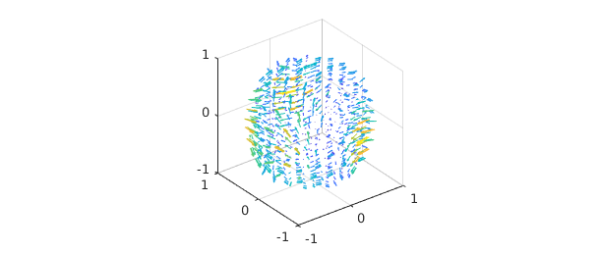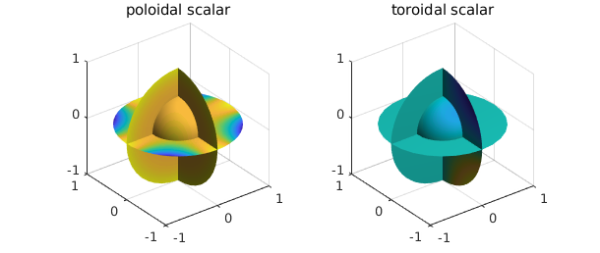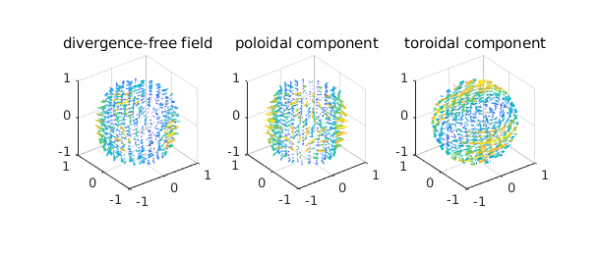### The poloidal-toroidal decomposition

In vector calculus, the poloidal-toroidal (PT) decomposition  is a restricted form of the Helmholtz-Hodge decomposition . It states that any sufficiently smooth and divergence-free vector field in the ball can be expressed as the sum of a poloidal field and a toroidal field. It is used in the analysis of divergence-free vector fields in geomagnetism, flow visualization, and incompressible fluid simulations.

For a given unit vector $\hat{r}$, a toroidal field, $\mathbf{T}$, is one that is orthogonal to $\hat{r}$, while a poloidal field, $\mathbf{P}$, is one whose curl is orthogonal to $\hat{r}$, i.e., $$\hat{r}\cdot \mathbf{T} = 0, \quad \hat{r}\cdot (\nabla \times \mathbf{P}) = 0.$$ In this setting, the natural unit vector $\hat{r}$ to select is the unit radial vector pointing away from the origin.

### The PT decomposition for vector fields in the unit ball

Let $w$ be a divergence-free vector field in the unit ball. Then the PT decomposition says that $w$ can be written as the sum $$w = \nabla\times\nabla\times(r P_w\hat{r}) + \nabla\times(rT_w\hat{r}),$$ where $P_w$ and $T_w$ are scalar-valued potential functions (called the poloidal and toroidal scalars). The scalars $P_w$ and $T_w$ are unique up to the addition of an arbitrary function that only depends on $r$.

In this Example, we show how this decomposition is computed by Ballfun and introduce the command PTdecomposition .

To illustrate the decomposition, we take the following divergence-free vector field:

Pw = ballfun(@(x,y,z)cos(x.*y));
Tw = ballfun(@(x,y,z)sin(y.*z));
w = ballfunv.PT2ballfunv(Pw, Tw);
quiver( w )We start by checking that $w$ is divergence-free:

norm( div( w ) )
ans =
4.162099210310871e-10


### Computing the PT decomposition

The scalars $P_w$ and $T_w$ are unique up to the addition of an arbitrary function that depends only on $r$. A popular additional constraint is to select $P_w$ and $T_w$ so that their integrals over the unit sphere are zero. Under this additional restriction, they satisfy the relations  $$\nabla_1^2 P_w = -rv_r,$$ $$\nabla_1^2 T_w = -\Lambda_1\cdot w,$$ where $\nabla_1^2$ and $\Lambda_1$ denote the dimensionless Laplacian and surface curl, respectively. These operations are defined in the spherical coordinates $(r,\lambda,\theta)$ by $$\nabla_1^2 = \frac{1}{\sin\theta}\partial_\theta(\sin\theta\partial_\theta) +\frac{1}{\sin^2\theta}\partial_\lambda^2,$$ $$\Lambda_1\cdot w = \frac{1}{\sin\theta}[\partial_\theta(w_\lambda\sin\theta) -\partial_\lambda w_\theta].$$ These constraints are imposed in the solver by requiring that the zeroth Fourier modes in $\lambda$ and $\theta$ are zero. This actually means that if $P_w$ is represented by a Chebyshev-Fourier-Fourier series, i.e., $$\sum_{i=0}^n\sum_{j=-n/2}^{n/2}\sum_{k=-n/2}^{n/2}a_{i,j,k}T_i(r)e^{\mathbf{i}j\lambda}e^{\mathbf{i}k\theta},$$ then $a_{i,0,0} = 0$ for $0\leq i\leq n$.

### The PTdecomposition command

Ballfun has a command PTdecomposition that computes the PT decomposition of a divergence-free vector field. The decomposition of $v$ can be computed as follows:

[Pw, Tw] = PTdecomposition( w );
subplot(1,2,1)
plot(Pw), title('poloidal scalar')
subplot(1,2,2)
plot(Tw), title('toroidal scalar')### Visualizing the decomposition

Here are plots of the decomposition.

[P,T] = ballfunv.PT2ballfunv(Pw,Tw);
subplot(1,3,1)
quiver( w ), title('divergence-free field')
subplot(1,3,2)
quiver( P ), title('poloidal component')
subplot(1,3,3)
quiver( T ), title('toroidal component')### Recovering the vector field from the PT scalars

The original vector field can be recovered from the poloidal and toroidal scalars since $$w = \nabla\times\nabla\times(rP_w\hat{r}) + \nabla\times(rT_w\hat{r}).$$ This operation is implemented in Ballfun in the PT2ballfunv command:

v = ballfunv.PT2ballfunv(Pw, Tw);

As a sanity check, we confirm that the decomposition has been successful:

norm( v - w )
ans =
1.281358965881723e-12


 G. Backus, Poloidal and toroidal fields in geomagnetic field modelling, Reviews of Geophysics, 24 (1986), pp. 75-109.

 N. Boullé and A. Townsend, Computing with functions on the ball, in preparation.

 Y. Tong, S. Lombeyda, A. Hirani, and M. Desbrun, Discrete multiscale vector field decomposition, ACM Trans. Graphics, 22 (2003), pp. 445-452.